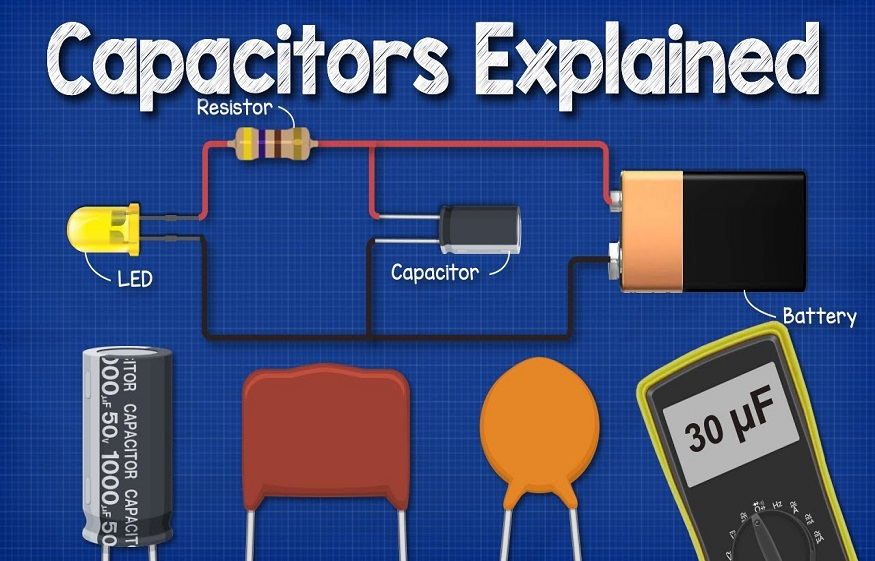# How to use a Capacitor in a Circuit?532 Views

Capacitors are a widely used electronic component, and it would be fair to say that we rarely find circuits without capacitors. This article will help you understand what a capacitor is, its working and how to use it in a circuit.

## What is Capacitor?

A capacitor is a two-terminal passive component capable of storing electric charges in it, and as a result, it develops a voltage across its terminals. We call the ability of a capacitor to store charges “capacitance.” The construction of a capacitor is simple. It consists of two conductive plates separated by a small distance; a dielectric is placed between the gap (dielectric is an insulator that is a poor conductor of electricity.) The area of the plate and the distance between the separation affect the capacitance of a capacitor. The capacitance of a capacitor is given by the formula C = Q/V, where C is the capacitance of a capacitor, Q is the amount of charge, and V is the voltage across it.

## How does a Capacitor work?

When we connect a DC source to the capacitor, one of the plates (Plate 1) is connected to the battery’s positive terminal, and the other (Plate 2) is connected to the battery’s negative terminal. When the potential of the battery is applied across the capacitor, Plate 1 becomes positive with respect to plate 2. At the steady-state condition, the current tries to flow from the positive plate to its negative plate. But it is unable to flow due to the dielectric material present between them. As a result, an electric field appears across the capacitor. After a point, the capacitor holds the maximum charge. This period of charging is called the charging time of the capacitor. When the battery is removed from the capacitor, the two plates hold a negative and positive charge for a specific time. Thus, the capacitor acts as a source of electrical energy. When the plates are connected to a load, the current flows to the load from Plate I to Plate II until all the charges are dissipated from both plates. This period of dissipation is known as the discharging time of the capacitor.

## Series and Parallel Connections of Capacitors

Several capacitors may be connected in a variety of applications.

## Series Connection of Capacitors

When capacitors are connected in series, the charge on each capacitor is the same; however, the voltage across them may be different.

When capacitors are connected in series, the equivalent capacitance is calculated using the following formula:

1/Ceq= 1/C1+ 1C2+…..+ 1/Cn

## Parallel Connection of Capacitors

When capacitors are connected in parallel, the potential difference V across each is the same, and the charge on each capacitor is different.

When capacitors are connected in parallel, the equivalent capacitance of capacitors in parallel is calculated using the following formula:

Ceq = C1 + C2 + C3….+ Cn

We use different combinations of capacitors to create any capacitance value that you might need in an electronic circuit.